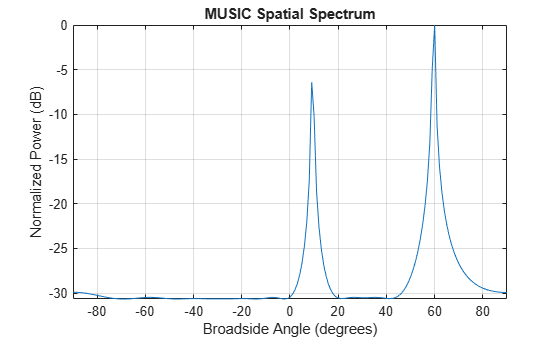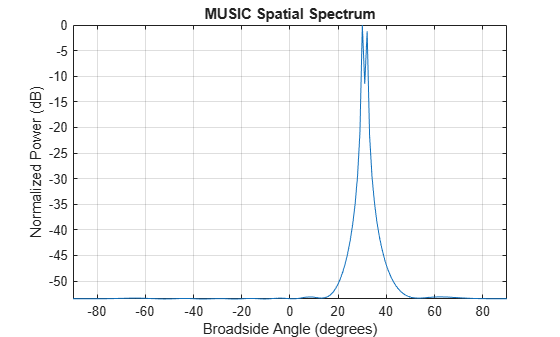# step

System object: phased.MUSICEstimator
Package: phased

Estimate direction of arrival using MUSIC

## Syntax

```spectrum = step(estimator,X) [spectrum,doa] = step(estimator,X) ```

## Description

Note

Instead of using the `step` method to perform the operation defined by the System object™, you can call the object with arguments, as if it were a function. For example, ```y = step(obj,x)``` and `y = obj(x)` perform equivalent operations.

`spectrum = step(estimator,X)` returns the MUSIC spectrum for a signal specified by `X`.

```[spectrum,doa] = step(estimator,X)``` also returns the signal broadside directions of arrival, `doa`. To use this syntax, set the `DOAOutputPort` property to `true`.

Note

The object performs an initialization the first time the object is executed. This initialization locks nontunable properties and input specifications, such as dimensions, complexity, and data type of the input data. If you change a nontunable property or an input specification, the System object issues an error. To change nontunable properties or inputs, you must first call the `release` method to unlock the object.

## Input Arguments

expand all

MUSIC estimator, specified as a `phased.MUSICEstimator` System object.

Example: `phased.MUSICEstimator`

Received signal, specified as an M-by-N complex-valued matrix. The quantity M is the number of sample values (snapshots) contained in the signal, and N is the number of sensor elements in the array.

The size of the first dimension of the input matrix can vary to simulate a changing signal length. A size change can occur, for example, in the case of a pulse waveform with variable pulse repetition frequency.

Example: `[[0;1;2;3;4;3;2;1;0],[1;2;3;4;3;2;1;0;0]]`

Data Types: `double`
Complex Number Support: Yes

## Output Arguments

expand all

MUSIC spatial spectrum, returned as a non-negative, real-valued K-length column vector representing the magnitude of the estimated MUSIC spatial spectrum. Each entry corresponds to an angle specified by the `ScanAngles` property.

Directions of arrival of the signals, returned as a real-valued L-length row vector. The direction of arrival angle is the angle between the source direction and the array axis or broadside angle. Angle units are in degrees. L is the number of signals specified by the `NumSignals` property or computed using the method specified by the `NumSignalsMethod` property.

#### Dependencies

To enable this output argument, set the `DOAOutputPort` property to `true`.

## Examples

expand all

Estimate the DOAs of two signals received by a standard 10-element ULA having an element spacing of 1 meter. Then plot the MUSIC spectrum.

Note: You can replace each call to the function with the equivalent `step` syntax. For example, replace `myObject(x)` with `step(myObject,x)`.

Create the ULA array. The antenna operating frequency is 150 MHz.

```fc = 150.0e6; array = phased.ULA('NumElements',10,'ElementSpacing',1.0);```

Create the arriving signals at the ULA. The true direction of arrival of the first signal is 10° in azimuth and 20° in elevation. The direction of the second signal is 60° in azimuth and -5° in elevation.

```fs = 8000.0; t = (0:1/fs:1).'; sig1 = cos(2*pi*t*300.0); sig2 = cos(2*pi*t*400.0); sig = collectPlaneWave(array,[sig1 sig2],[10 20; 60 -5]',fc); noise = 0.1*(randn(size(sig)) + 1i*randn(size(sig)));```

Estimate the DOAs.

```estimator = phased.MUSICEstimator('SensorArray',array,... 'OperatingFrequency',fc,... 'DOAOutputPort',true,'NumSignalsSource','Property',... 'NumSignals',2); [y,doas] = estimator(sig + noise); doas = broadside2az(sort(doas),[20 -5])```
```doas = 1×2 9.5829 60.3813 ```

Plot the MUSIC spectrum.

`plotSpectrum(estimator,'NormalizeResponse',true)`First, estimate the DOAs of two signals received by a standard 10-element ULA having an element spacing of one-half wavelength.Then, plot the spatial spectrum.

Note: You can replace each call to the function with the equivalent `step` syntax. For example, replace `myObject(x)` with `step(myObject,x)`.

The antenna operating frequency is 150 MHz. The arrival directions of the two signals are separated by 2°. The direction of the first signal is 30° azimuth and 0° elevation. The direction of the second signal is 32° azimuth and 0° elevation. Estimate the number of signals using the Minimum Description Length (MDL) criterion.

Create the signals arriving at the ULA.

```fs = 8000; t = (0:1/fs:1).'; f1 = 300.0; f2 = 600.0; sig1 = cos(2*pi*t*f1); sig2 = cos(2*pi*t*f2); fc = 150.0e6; c = physconst('LightSpeed'); lam = c/fc; array = phased.ULA('NumElements',10,'ElementSpacing',0.5*lam); sig = collectPlaneWave(array,[sig1 sig2],[30 0; 32 0]',fc); noise = 0.1*(randn(size(sig)) + 1i*randn(size(sig)));```

Estimate the DOAs.

```estimator = phased.MUSICEstimator('SensorArray',array,... 'OperatingFrequency',fc,'DOAOutputPort',true,... 'NumSignalsSource','Auto','NumSignalsMethod','MDL'); [y,doas] = estimator(sig + noise); doas = broadside2az(sort(doas),[0 0])```
```doas = 1×2 30.0000 32.0000 ```

Plot the MUSIC spectrum.

`plotSpectrum(estimator,'NormalizeResponse',true)`## Version History

Introduced in R2016b